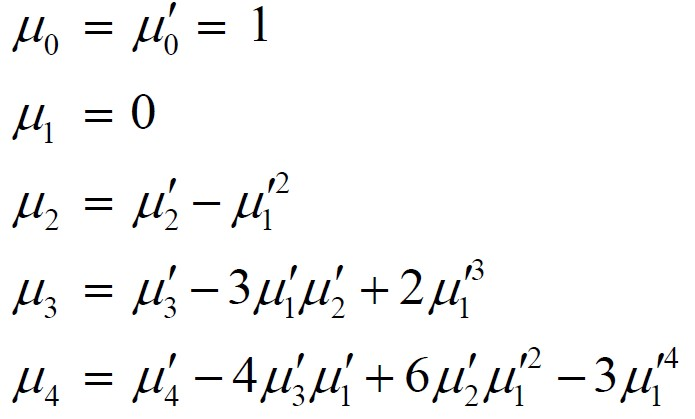Related Articles
ML | Raw and Central Moments
• Difficulty Level : Expert
• Last Updated : 26 Jul, 2019

Moments are a set of statistical parameters which are used to describe different characteristics and feature of a frequency distribution i.e. central tendency, dispersion, symmetry, and peakedness (hump) of the frequency curve.

For Ungrouped data i.e. discrete data, observations on a variable X are obtained as, For Grouped data i.e. continuous data, observations on a variable X are obtained and tabulated in K class intervals in a frequency table. The mid points of the inervals are denoted bywhich occur with frequenciesrespectively and.

Class IntervalsMid Points ()Absolute Frequency ()Moments about an arbitrary point A
Themoment of a variable X about any arbitrary point A on the observationsis defined as:

For ungrouped dataFor grouped datawhereMoment about any arbitrary point in Python –

Consider the given data points. Following are the time (in hours) spent by 20 different persons at GeeksforGeeks portal every week.

15, 25, 18, 36, 40, 28, 30, 32, 23, 22, 21, 27, 31, 20, 14, 10, 33, 11, 7, 13
 # data pointstime = [15, 25, 18, 36, 40, 28, 30, 32, 23, 22,         21, 27, 31, 20, 14, 10, 33, 11, 7, 13]  # Arbitrary point A = 22  # Moment for r = 1moment = (sum([(item-A) for item in time]))/len(time)

## Raw Moments –

Themoment around origin A = 0 known as raw moment and is defined as:

For ungrouped data,For grouped data,where,Notes:

-> We can find first raw moment () just by replacing r with 1 and second raw moment () just by replacing r with 2 and so on.
-> When r = 0 the momentfor both grouped and ungrouped data.

Raw moment in Python –

 # data pointstime = [15, 25, 18, 36, 40, 28, 30, 32, 23,       22, 21, 27, 31, 20, 14, 10, 33, 11, 7, 13]    # Moment for r = 1moment = sum(time)/len(time)

## Central Moments –

The moments of a variable X about the arithmetic mean () are known as central moments and defined as:

For ungrouped data,For grouped data,whereandNotes:

-> We can find first raw moment () just by replacing r with 1 and second raw moment () just by replacing r with 2 and so on.
-> When r = 0 the moment, and when r = 1 the momentfor both grouped and ungrouped data.

 # data pointstime = [15, 25, 18, 36, 40, 28, 30, 32, 23, 22,       21, 27, 31, 20, 14, 10, 33, 11, 7, 13]  # Mean A = sum(time)/len(time)  # Moment for r = 1moment = (sum([(item-A) for item in time]))/len(time)

Relationship between Raw and Central moments –My Personal Notes arrow_drop_up